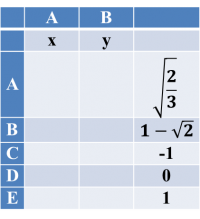## Another fresh question on 2 Part- Quadratic function

### GMAT/MBA Expert

GMAT Instructor
Posts: 645
Joined: 26 Jul 2010
Location: US
Thanked: 527 times
Followed by:227 members

### Another fresh question on 2 Part- Quadratic function

by e-GMAT » Fri Jan 25, 2013 4:16 am
Try yet another 2 part question- fresh from theÂ e-GMATÂ bakery!

A function f(x, y) is such that f(x,y)=3x^2-2xy+y^2+4. Select one value for x, & one value for y such that given information implies that f(x, y) = 8. Make only two selections, one in each column.Want to view similar 2 part questions with an interactive audio visual solution? Register here at e-GMAT.

Don't forget to attend GMAT strategy session by e-GMAT CEO Rajat Sadana on 26th January. Pl. click link below to know more.

-Shalabh

Legendary Member
Posts: 1404
Joined: 20 May 2008
Thanked: 18 times
Followed by:2 members
by tanviet » Thu Feb 07, 2013 11:37 pm
how to pick the number

from where do we begin?

### GMAT/MBA Expert

GMAT Instructor
Posts: 645
Joined: 26 Jul 2010
Location: US
Thanked: 527 times
Followed by:227 members

### Another fresh question on 2 Part- Quadratic function

by e-GMAT » Fri Feb 08, 2013 12:33 am
e-GMAT wrote:Try yet another 2 part question- fresh from theÂ e-GMATÂ bakery!

A function f(x, y) is such that f(x,y)=3x^2-2xy+y^2+4. Select one value for x, & one value for y such that given information implies that f(x, y) = 8. Make only two selections, one in each column.Want to view similar 2 part questions with an interactive audio visual solution? Register here at e-GMAT.

-Shalabh
Hi,

When we look at the question and then at the options, we find that plugging values is the best way to approach this question.
There are 3 things that should be kept in mind while picking option values.

1.Pick integers first. They are easy to work on.
There are 3 values in the option list, which are integers.

2.Pick '0' first. This will eliminate one variable completely for computation.

3.What to choose first; x or y? One should always observe right hand side of the function. If number of terms of x is more than the number of terms of y, then plug in the option value in x first, and vice versa.

We choose the values for x in the order of 0, 1, and -1 to plug in.

Now, we plug in the value of f(x, y) =8, & x=0 in the equation, and we get,

8=3.(0)^2- 2.0.y + y^2 + 4
8=y^2 + 4
4=y^2

y= Â±2

This means for x=0, y is either 2 or -2. There is no such option available for values: 2 or-2 , hence these pair of values cannot be correct.

Now, we try with x= 1.

Now, we plug in the value of f(x, y) =8, & x=1 in the equation, so we get,
8=3.(1)^2-2.1.y+y^2+4
8=3-2y+y^2+4
1= -2y + y^2
y^2-2y-1=0

This is a quadratic equation. We know the formula of quadratic equation- ay^2+by+c=0 as
y=(-bÂ±âˆš(b^2-4.a.c))/2a, so apply this formula to get the value of y.

Here a=1, b=-2, & c=-1

y = (2Â±âˆš(ã€–(-2)ã€—^2-4.1.-1))/2 = (2Â±âˆš8)/2= (2Â±2âˆš2)/2 = 1Â±âˆš2

This means for x=1, y is either 1+âˆš2 or 1-âˆš2

Since x = 1, & y =1-âˆš2 is available in options, hence answers are E, & B.

Hope it helps!

-Shalabh

Senior | Next Rank: 100 Posts
Posts: 45
Joined: 06 Apr 2012
Thanked: 3 times
Followed by:2 members
GMAT Score:500
by BTG14 » Mon Feb 25, 2013 6:06 am
Good one..• Page 1 of 1